## The Binomial Formula

The binomial distribution is a discrete probability distribution of the successes in a sequence of $\text{n}$ independent yes/no experiments.

### Learning Objectives

Employ the probability mass function to determine the probability of success in a given amount of trials

### Key Takeaways

#### Key Points

• The probability of getting exactly $\text{k}$ successes in $\text{n}$ trials is given by the Probability Mass Function.
• The binomial distribution is frequently used to model the number of successes in a sample of size $\text{n}$ drawn with replacement from a population of size $\text{N}$.
• The binomial distribution is the discrete probability distribution of the number of successes in a sequence of $\text{n}$ independent yes/no experiments, each of which yields success with probability $\text{p}$.

#### Key Terms

• central limit theorem: a theorem which states that, given certain conditions, the mean of a sufficiently large number of independent random variables–each with a well-defined mean and well-defined variance– will be approximately normally distributed
• probability mass function: a function that gives the probability that a discrete random variable is exactly equal to some value

In probability theory and statistics, the binomial distribution is the discrete probability distribution of the number of successes in a sequence of $\text{n}$ independent yes/no experiments, each of which yields success with probability $\text{p}$. The binomial distribution is the basis for the popular binomial test of statistical significance.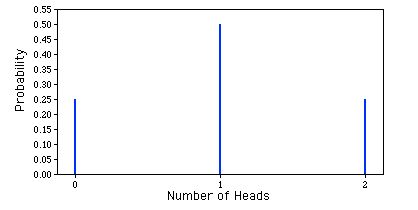Binomial Probability Distribution: This is a graphic representation of a binomial probability distribution.

The binomial distribution is frequently used to model the number of successes in a sample of size $\text{n}$ drawn with replacement from a population of size $\text{N}$. If the sampling is carried out without replacement, the draws are not independent and so the resulting distribution is a hypergeometric distribution, not a binomial one. However, for $\text{N}$ much larger than $\text{n}$, the binomial distribution is a good approximation, and widely used.

In general, if the random variable $\text{X}$ follows the binomial distribution with parameters $\text{n}$ and $\text{p}$, we write $\text{X} \sim \text{B}(\text{n}, \text{p})$. The probability of getting exactly $\text{k}$ successes in $\text{n}$ trials is given by the Probability Mass Function:

$\displaystyle \text{f}(\text{k}; \text{n}, \text{p}) = \text{P}(\text{X}=\text{k}) = {{\text{n}}\choose{\text{k}}}\text{p}^\text{k}(1-\text{p})^{\text{n}-\text{k}}$

For $\text{k} = 0, 1, 2, \dots, \text{n}$ where:

$\displaystyle {{\text{n}}\choose{\text{k}}} = \frac{\text{n}!}{\text{k}!(\text{n}-\text{k})!}$

Is the binomial coefficient (hence the name of the distribution) “n choose k, also denoted $\text{C}(\text{n}, \text{k})$ or $_\text{n}\text{C}_\text{k}$. The formula can be understood as follows: We want $\text{k}$ successes ($\text{p}^\text{k}$) and $\text{n}-\text{k}$ failures ($(1-\text{p})^{\text{n}-\text{k}}$); however, the $\text{k}$ successes can occur anywhere among the $\text{n}$ trials, and there are $\text{C}(\text{n}, \text{k})$ different ways of distributing $\text{k}$ successes in a sequence of $\text{n}$ trials.

One straightforward way to simulate a binomial random variable $\text{X}$ is to compute the sum of $\text{n}$ independent 0−1 random variables, each of which takes on the value 1 with probability $\text{p}$. This method requires $\text{n}$ calls to a random number generator to obtain one value of the random variable. When $\text{n}$ is relatively large (say at least 30), the Central Limit Theorem implies that the binomial distribution is well-approximated by the corresponding normal density function with parameters $\mu = \text{np}$ and $\sigma = \sqrt{\text{npq}}$.

### Figures from the Example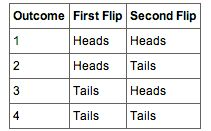Table 1: These are the four possible outcomes from flipping a coin twice.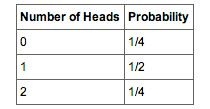Table 2: These are the probabilities of the 2 coin flips.

## Binomial Probability Distributions

This chapter explores Bernoulli experiments and the probability distributions of binomial random variables.

### Learning Objectives

Apply Bernoulli distribution in determining success of an experiment

### Key Takeaways

#### Key Points

• A Bernoulli (success-failure) experiment is performed $\text{n}$ times, and the trials are independent.
• The probability of success on each trial is a constant $\text{p}$; the probability of failure is $\text{q}=1-\text{p}$.
• The random variable $\text{X}$ counts the number of successes in the $\text{n}$ trials.

#### Key Terms

• Bernoulli Trial: an experiment whose outcome is random and can be either of two possible outcomes, “success” or “failure”

Many random experiments include counting the number of successes in a series of a fixed number of independently repeated trials, which may result in either success or failure. The distribution of the number of successes is a binomial distribution. It is a discrete probability distribution with two parameters, traditionally indicated by $\text{n}$, the number of trials, and $\text{p}$, the probability of success. Such a success/failure experiment is also called a Bernoulli experiment, or Bernoulli trial; when $\text{n}=1$, the Bernoulli distribution is a binomial distribution.

Named after Jacob Bernoulli, who studied them extensively in the 1600s, a well known example of such an experiment is the repeated tossing of a coin and counting the number of times “heads” comes up.

In a sequence of Bernoulli trials, we are often interested in the total number of successes and not in the order of their occurrence. If we let the random variable $\text{X}$ equal the number of observed successes in $\text{n}$ Bernoulli trials, the possible values of $\text{X}$ are $0, 1, 2, \dots, \text{n}$. If $\text{x}$ success occur, where $\text{x}=0, 1, 2, \dots, \text{n}$, then $\text{n}-\text{x}$ failures occur. The number of ways of selecting $\text{x}$ positions for the $\text{x}$ successes in the $\text{x}$ trials is:

$\displaystyle {{\text{n}}\choose{\text{x}}} = \frac{\text{n}!}{\text{x}!(\text{n}-\text{x})!}$

Since the trials are independent and since the probabilities of success and failure on each trial are, respectively, $\text{p}$ and $\text{q}=1-\text{p}$, the probability of each of these ways is $\text{p}^\text{x}(1-\text{p})^{\text{n}-\text{x}}$. Thus, the p.d.f. of $\text{X}$, say $\text{f}(\text{x})$, is the sum of the probabilities of these ($\text{nx}$) mutually exclusive events–that is,

$\text{f}(\text{x})=(\text{nx})\text{p}^\text{x}(1-\text{p})^{\text{n}-\text{x}}$

$\text{x}=0, 1, 2, \dots, \text{n}$

These probabilities are called binomial probabilities, and the random variable $\text{X}$ is said to have a binomial distribution.Wind pollination: These male (a) and female (b) catkins from the goat willow tree (Salix caprea) have structures that are light and feathery to better disperse and catch the wind-blown pollen.Probability Mass Function: A graph of binomial probability distributions that vary according to their corresponding values for $\text{n}$ and $\text{p}$.

## Mean, Variance, and Standard Deviation of the Binomial Distribution

In this section, we’ll examine the mean, variance, and standard deviation of the binomial distribution.

### Learning Objectives

Examine the different properties of binomial distributions

### Key Takeaways

#### Key Points

• The mean of a binomial distribution with parameters $\text{N}$ (the number of trials) and $\text{p}$ (the probability of success for each trial) is $\text{m}=\text{Np}$.
• The variance of the binomial distribution is $\text{s}^2 = \text{Np}(1-\text{p})$, where $\text{s}^2$ is the variance of the binomial distribution.
• The standard deviation ($\text{s}$) is the square root of the variance ($\text{s}^2$).

#### Key Terms

• variance: a measure of how far a set of numbers is spread out
• mean: one measure of the central tendency either of a probability distribution or of the random variable characterized by that distribution
• standard deviation: shows how much variation or dispersion exists from the average (mean), or expected value

As with most probability distributions, examining the different properties of binomial distributions is important to truly understanding the implications of them. The mean, variance, and standard deviation are three of the most useful and informative properties to explore. In this next section we’ll take a look at these different properties and how they are helpful in establishing the usefulness of statistical distributions. The easiest way to understand the mean, variance, and standard deviation of the binomial distribution is to use a real life example.

Consider a coin-tossing experiment in which you tossed a coin 12 times and recorded the number of heads. If you performed this experiment over and over again, what would the mean number of heads be? On average, you would expect half the coin tosses to come up heads. Therefore, the mean number of heads would be 6. In general, the mean of a binomial distribution with parameters $\text{N}$ (the number of trials) and $\text{p}$ (the probability of success for each trial) is:

$\text{m}=\text{Np}$

Where $\text{m}$ is the mean of the binomial distribution.

The variance of the binomial distribution is:

$\text{s}^2 = \text{Np}(1-\text{p})$, where $\text{s}^2$ is the variance of the binomial distribution.

The coin was tossed 12 times, so $\text{N}=12$. A coin has a probability of 0.5 of coming up heads. Therefore, $\text{p}=0.5$. The mean and standard deviation can therefore be computed as follows:

$\text{m}=\text{Np}=12\cdot0.5 = 6$

$\text{s}^2=\text{Np}(1-\text{p})=12\cdot0.5\cdot(1.0-0.5)=3.0$

Naturally, the standard deviation ($\text{s}$) is the square root of the variance ($\text{s}^2$).Coin Flip: Coin flip experiments are a great way to understand the properties of binomial distributions.

## Additional Properties of the Binomial Distribution

In this section, we’ll look at the median, mode, and covariance of the binomial distribution.

### Learning Objectives

Explain some results of finding the median in binomial distribution

### Key Takeaways

#### Key Points

• There is no single formula for finding the median of a binomial distribution.
• The mode of a binomial $\text{B}(\text{n}, \text{p})$ distribution is equal to.
• If two binomially distributed random variables $\text{X}$ and $\text{Y}$ are observed together, estimating their covariance can be useful.

#### Key Terms

• floor function: maps a real number to the smallest following integer
• covariance: A measure of how much two random variables change together.
• median: the numerical value separating the higher half of a data sample, a population, or a probability distribution, from the lower half
• Mode: the value that appears most often in a set of data

In general, there is no single formula for finding the median of a binomial distribution, and it may even be non-unique. However, several special results have been established:

If $\text{np}$ is an integer, then the mean, median, and mode coincide and equal $\text{np}$.

Any median $\text{m}$ must lie within the interval $\lfloor \text{np}\rfloor \leq \text{m} \leq \lceil \text{np}\rceil$.

A median $\text{m}$ cannot lie too far away from the mean: $|\text{m} \text{np}| \leq \min { \ln { 2 } },\max { (\text{p},1 - \text{p} )}$.

The median is unique and equal to $\text{m} = round(\text{np})$ in cases where either $\text{p} \leq 1 \ln 2$ or $\text{p} \geq \ln 2$ or $|\text{m} \text{np}| \leq \min{(\text{p}, 1 \text{p})}$ (except for the case when $\text{p} = \frac{1}{2}$ and n is odd ).

When$\text{p} = \frac{1}{2}$ and n is odd, any number m in the interval $\frac{1}{2} \cdot (\text{n} 1) \leq \text{m} \leq \frac{1}{2} \cdot (\text{n} + 1)$ is a median of the binomial distribution. If $\text{p} = \frac{1}{2}$ and n is even, then $\text{m} = \frac{n}{2}$ is the unique median.

There are also conditional binomials. If $\text{X} \sim \text{B}(\text{n}, \text{p})$ and, conditional on $\text{X}, \text{Y} \sim \text{B}(\text{X}, \text{q})$, then Y is a simple binomial variable with distribution.

The binomial distribution is a special case of the Poisson binomial distribution, which is a sum of n independent non-identical Bernoulli trials Bern(pi). If X has the Poisson binomial distribution with p1=…=pn=pp1=\ldots =pn=p then ∼B(n,p)\sim B(n, p).

Usually the mode of a binomial B(n, p) distribution is equal to where is the floor function. However, when $(\text{n} + 1)\text{p}$ is an integer and p is neither 0 nor 1, then the distribution has two modes: $(\text{n} + 1)\text{p}$ and $(\text{n} + 1)\text{p} 1$. When p is equal to 0 or 1, the mode will be 0 and n, respectively. These cases can be summarized as follows: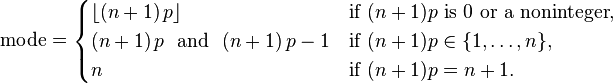Summary of Modes: This summarizes how to find the mode of a binomial distribution.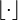Floor Function: Floor function is the lowest previous integer in a series.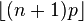Mode: This formula is for calculating the mode of a binomial distribution.

If two binomially distributed random variables X and Y are observed together, estimating their covariance can be useful. Using the definition of covariance, in the case n = 1 (thus being Bernoulli trials) we have.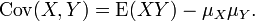Covariance 1: The first part of finding covariance.

The first term is non-zero only when both X and Y are one, and μX and μY are equal to the two probabilities. Defining pB as the probability of both happening at the same time, this gives and for n independent pairwise trials.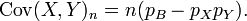Covariance 3: The final formula for the covariance of a binomial distribution.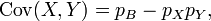Covariance 2: The next step in determining covariance.

If X and Y are the same variable, this reduces to the variance formula given above.